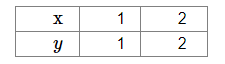# The graph of the linear equation x − y = 0 passes through the point

Question:

The graph of the linear equation x − y = 0 passes through the point

(a) $\left(\frac{-1}{2}, \frac{1}{2}\right)$

(b) $\left(\frac{3}{2}, \frac{-3}{2}\right)$

(c) $(0,-1)$

(d) $(1,1)$

Solution:

(d) (1, 1)

Given equation: $x-y=0$ or, $x=y$

When $x=1, y=1$

When $x=2, y=2 \ldots$ and so on

Thus, we get the following table:​Plot the points on the graph paper. Join the points and extend them in both the directions.

We can see the linear equation $x-y=0$ passes through the point $(1,1)$